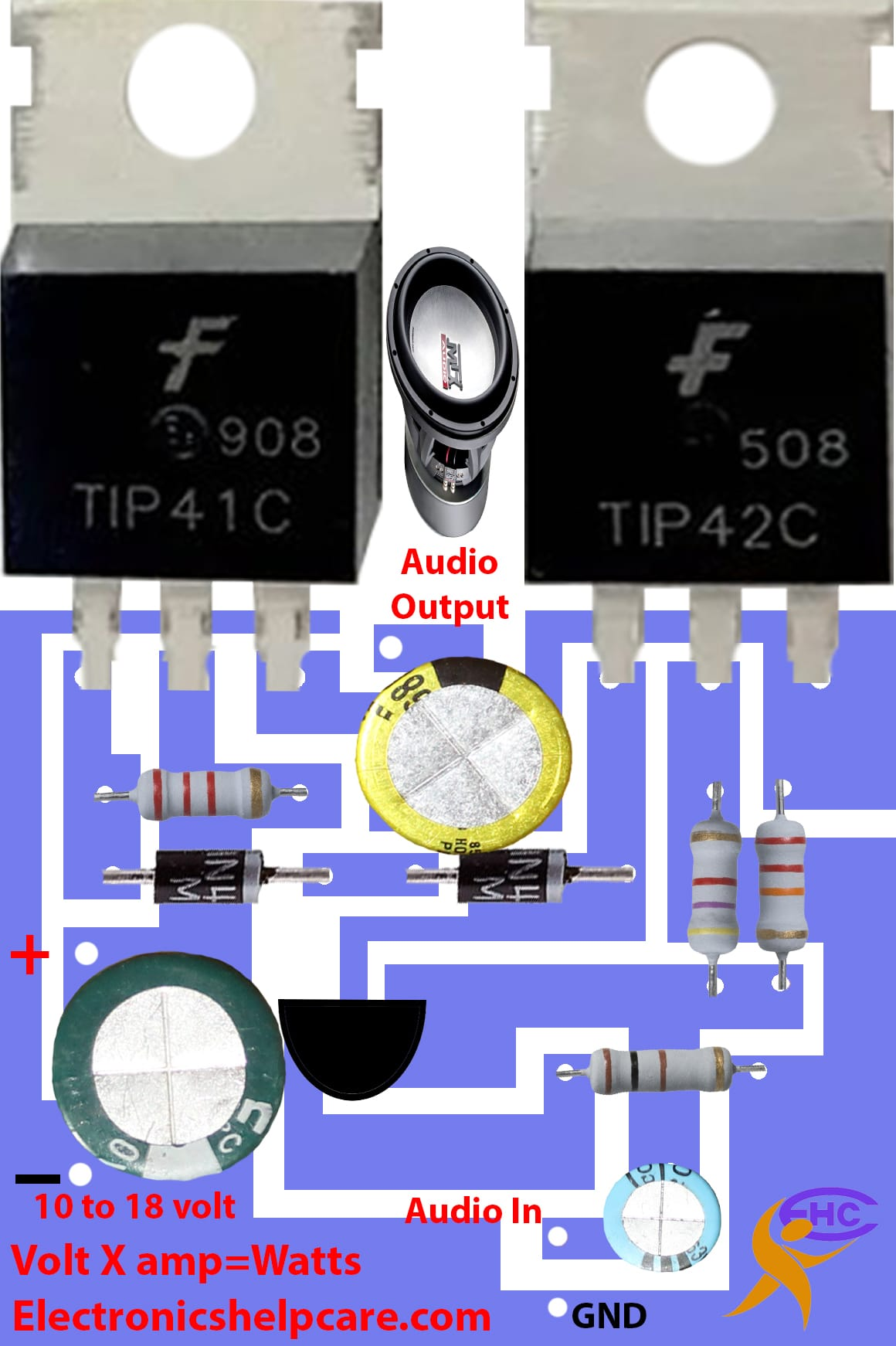# how to make 12 voltage amplifier circuit?

## 12 voltage amplifier using transistor TIP41 and TIP42

This is a mini amplifier circuit diagram. this circuit run from 10 voltage to 18 voltage. normally we can use 12 voltages. we can use 12 voltage battery also. here used 2 transistors only. tip41 is an NPN transistor and Tip42 is a PNP transistor. it’s one pair transistor that can make a maximum of 50 watts. it also depends on amplifier voltage and amperes.We know that Voltage X amperes = Watts.  so if we use 12 voltage and 2 amperes then we will get.  12 X 2 = 24 watts. or if we use 12 voltage 4 amperes then we get 12 X 3 = 36 watts. but we have to know we can’t use 4 amperes. because 2 transistor maximum can take 3 amperes. we can use 18 voltage 3 amperes. then 18 X 3 = 54 watts.If you need another amplifier circuit diagram please visit (Diagram)How to make an amplifier circuit diagram

We have another post for you. like repairing amplifiers,

If you like electronics please visit this site